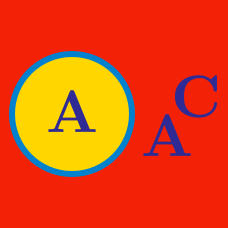Probability

# Complements: Level 2 Challenges

Three integers are chosen at random without replacement from the first 20 positive integers.

What is the probability that their product is even?

How many people must we have, in order for the probability that at least 1 person was born in a leap year to be greater than $50 \%$?

David is the leader of the David Committee. He wants to appoint 3 people to be on the Head Council. He has to choose from 9 applicants, three of whom are Tommy, Jack, and Michael. In how many ways can he choose the people to be on the Council, so that at least one of Tommy, Jack, and Michael is chosen?

Five distinct numbers are randomly chosen from the set $S = \{0,1,2,3,4,5,6,7,8,9\}$ and multiplied. The likelihood that the product is even can be written as $\frac{a}{b}$, where $a, b$ are relatively prime positive integers. Find $a + b$.Trevor and I were playing golf.

As we all know, Trevor is a pro golfer and I don't know squat about golf (except the fact that we are supposed to hit golf balls with a club).

Now, he made a bet with me. He said, "We will play $N$ matches where you're gonna choose the value of $N$. If you win at least one match, I'll pay you $\100$ since I'm super rich and if you don't, you'll pay me $\1$"

Now, I opened my wallet and I saw that it was emptier than a banker's heart. Amongst the cobwebs and flies in it, luckily, there was a $\1$ bill. Naturally, I accepted the bet since I'm almost broke and need cash.

Generally, if I were to play a match against him, I would have a probability of $0$ of winning. But since here's money involved, the probability of me winning a single match against him is boosted to $\dfrac{1}{3}$.

I want to have at least a $90\%$ chance of winning the bet. What is the minimum value of $N$ that I should choose?

×